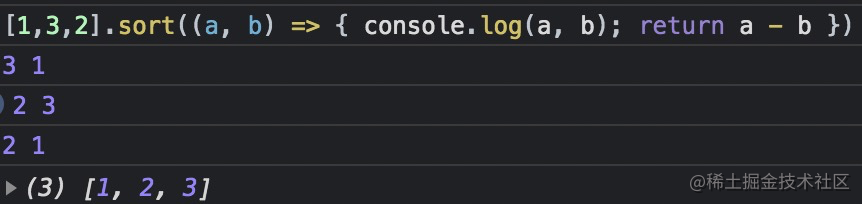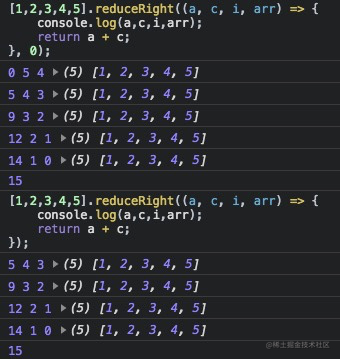# 1. 起因# 2. 场景

## 2.1. `sort` 的参数与规则

``````[1, 3, 2].sort((a, b) => a - b);
[1, 3, 2].sort((a, b) => b - a);

`a - b``b - a` 哪个是从小到大?

`MDN` 上的定义来看

• 如果 `a - b < 0``a``b` 的前面
• 如果 `a - b > 0``b``a` 的前面
• 如果 `a - b == 0``a``b` 可以认为不会交换`a - b` 为例

`3 - 1` > 0 及 `a - b` > 0 , 所以 `b` 应该在 `a` 的前面

PS: 感谢 @shyg 的指正，以上仅代表了 `chrome` 浏览器，部分浏览器参数的逻辑为下表，有兴趣的小伙伴可以自己去试试相应的浏览器～

chromeb 是前面的元素，a 是后面的元素
firefoxa 是前面的元素，b 是后面的元素
safari同 chrome
Microsoft Edge同 chrome

`sort` 方法还有一个注意点是虽然函数的返回值是一个排列好的数据。但是原数组已经发生了改变，在实际使用中一定要注意，避免出现以下情况！

``````var arr = [1, 2, 3];
var arr1 = arr.sort((a, b) => b - a);

// 然后以为 arr 还是原数组的情况 ～

## 2.2. 在 `for` 循环或 `forEach` 中 `return`

`for` 循环中 `return ` 确实可以跳出循环并返回结果

`forEach` 却并没有这个功效

``````var array = [1, 2, 3, 4, 5];

// 假设需要实现一个 get 方法，获取数组中第一个大于 3 的数字
function get(arr) {
// 可以这么写 ✅
for (let i of arr) {
if (i >= 3) {
return i;
}
}
return null;

// 错误 ❌
arr.forEach((item) => {
if (item >= 3) {
return item;
}
});
return null;

// 错误 ❌
const result = arr.forEach((item) => {
if (item >= 3) {
return item;
}

return null;
});
return result;
}

## 2.3. 更准确的 `includes`

`includes` 可以判断数组中是否包含 `NAN`，但是 `indexOf` 不行

## 2.4. 容易遗忘的 `unshift`

``````.concat([2, 3]);

[1, ...[2,3]]

## 2.5. `reduce` 的孪生兄弟

`api` 时发现有一个 `reduceRight` 是从后往前执行 `reduce` 的可以当成 `[].reverse().reduce` 使用。• 上面的 `reduceRight` 首次遍历，当前项是 `5` ，所以输出的下标是 `4`
• 下面没有加默认值的 `reduceRight` 首次遍历，当前项是 `4`, 所以输出的下标是 `3`

## 2.6. Mock数据高手 `fill`

``````Array(5).fill(1); // [1, 1, 1, 1, 1]

## 2.7. `for of` 的使用场景

``````// 第一种情况，找到数组中符合要求的一项
let arr = [];
let findItem = defaultValue;

// 正确 ✅
for (let item of arr) {
if (item === xxx) {
findItem = item;
break;
}
}

// 使用find也是可以的 ✅
const findItem = arr.find((item) => item === xxx) || defaultValue;

// 第二种情况，遍历数组，用里面的数据配合原生api组合调用
let arr = [];

// 例如使用数组元素，绘制canvas的场景
for (let item of arr) {
document.getElementById('canvas').drawImage(item.url, item.x, item.y, item.width, item.height)
}

## 2.8. `every` 与 `some`

`every` 需要数组中所有的项满足条件，才可返回 `true`

`some` 需要数组中只有一项满足条件，即可返回 `true`

``````// 他们均可以让以下场景的语义化更简洁

const arr = [100, 100, 100];
const arr1 = [100, 0, 0];

// for 循环写法
let isAllRight = true;
for (let item of arr) {
if (item !== 100) {
isAllRight = false;
break;
}
}

let hasAllRight = false;
for (let item of arr) {
if (item === 100) {
hasAllRight = true;
break;
}
}

// ✅ every 写法
const isAllRight = arr.every((item) => item === 100);

// ✅ some 写法
const hasAllRight = arr1.some((item) => item === 100);

// 如果数组是如上的简单结构，其实 some 可以用 includes/indexOf 代替
const hasAllRight = arr1.includes(100);
const hasAllRight = arr1.indexOf(100) > -1;

// 但是如果数组是 [{score: 100}, {score: 100}, {score: 0}] 对象格式，就需要使用 some